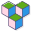CppBuzz# C++ Objective Questions - Page-14

Page 14 (261 - 280)

Question: 261

(A) Hello Derived
(B) Hello Base
(C) Hello Derived Hello Base
(D) Error: not matching func to call ‘derived::derived(cppbuzz_base&)’

Compiler
Level: Practitioner Category: typecasting
[Posted by: Admin | Chicago, USA]

Question: 262

(A) Both pointers hold same object
(B) They don't hold same object
(C) Compilation Error
(D) Exception occured

Compiler
Level: Practitioner Category: singleton
[Posted by: Admin | Chicago, USA]

Question: 263

(A)
(B)
(C)
(D)

Compiler
Level: Practitioner Category: singleton
[Posted by: Admin | Chicago, USA]

Question: 264

(A) error: cannot declare variable ‘obj’ to be of abstract type ‘cppbuzz’
(B) cppbuzz
(C) ~cppbuzz
(D) cppbuzz~cppbuzz

Compiler
Level: Practitioner Category: abstract_class
[Posted by: Admin | Chicago, USA]

Question: 265

(A) 4
(B) 8
(C) 12
(D) 16

Compiler
Level: Expert Category: abstract_class
[Posted by: Admin | Chicago, USA]

Question: 266

(A) True
(B) False

Compiler
Level: Practionar Category: virtual_func

Question: 267

(A) Compilation Error
(B) 10, 20
(C) 10, 10
(D) 10, Undfefined Value

Compiler
Level: Expert Category: classesAndobjects
[Posted by: Admin | Chicago, USA]

Question: 268

(A) O(log N)
(B) O(N/2)
(C) O(N)
(D) O(N^2)

Compiler
Level: Beginner Category: time_space_complexity
[Posted by: CppBuzz | Chicago, USA]

Question: 269

(A) Compilation error
(B) O(logN)
(C) O(N/2)
(D) O(N)

Compiler
Level: Practionar Category: time_space_complexity
[Posted by: CppBuzz | Chicago, USA]

Question: 270

(A) O(2N)
(B) O(N)
(C) O(N+N)
(D) O(N*N)

Compiler
Level: Practionar Category: time_space_complexity
[Posted by: CppBuzz | Chicago, USA]

Question: 271

(A) O(N+M)
(B) O(N*M)
(C) O(N)
(D) O(M)

Compiler
Level: Practionar Category: time_space_complexity
[Posted by: CppBuzz | Chicago, USA]

Question: 272

(A) O(N)
(B) O(Nlogn)
(C) O(N^2)
(D) O(N^3)

Compiler
Level: Practionar Category: time_space_complexity
[Posted by: CppBuzz | Chicago, USA]

Question: 273

(A) O(111*N)
(B) O(Nlogn)
(C) O(1)
(D) O(N)

Compiler
Level: Practionar Category: time_space_complexity
[Posted by: CppBuzz | Chicago, USA]

Question: 274

(A) O(N)
(B) O(logN)
(C) O(sqrt(N))
(D) O(∞)

Compiler
Level: Practionar Category: time_space_complexity
[Posted by: CppBuzz | Chicago, USA]

Question: 275

(A) O(LogN)
(B) O(1)
(C) O(N)
(D) O(N longN)

Compiler
Level: Practionar Category: time_space_complexity
[Posted by: CppBuzz | Chicago, USA]

Question: 276

(A) O(LogN) , O(N)
(B) O(N), O(N)
(C) O(N), O(1)
(D) O(N), O(0)

Compiler
Level: Practionar Category: time_space_complexity
[Posted by: CppBuzz | Chicago, USA]